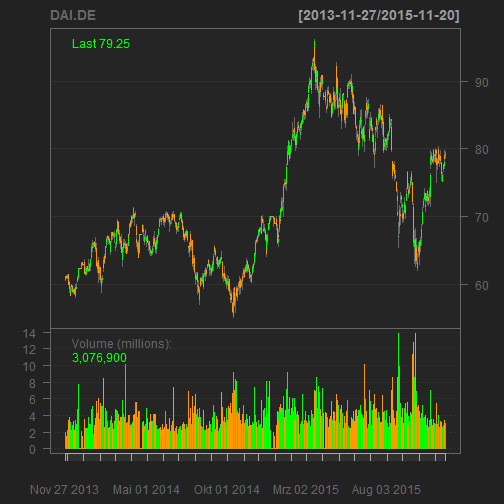# Revisiting barrier bond

In Analyse einer Barriere-Anleihe I was estimating the present value of a barrier bond emitted by Berliner Landesbank, which referred to the stock price of Daimler: The payout would be 100, if the price was either allways above the barrier of 70%, or, if it dipped below the barrier, but closed above the initial price at the maturity of the bond. In every other case, the buyer would get the value of the stock at maturity. For details on the bond, go here.

Using stochastic modelling on a 13 year history of the Daimler stock price, I estimated a present value at offering of 98.6% assuming a risk free rate of 2% for the 2 year maturity (I checked the sensitivity of the result to the risk free rate, but even at a -0.5% the mean present value is at 99.4%, so a slight loss).

Today I wanted to check what the actual payout would have been.

payoutEnd = function(basis, barriere, kurse)
{
basis = as.numeric(basis)
barriere = as.numeric(barriere)
kurse = as.numeric(kurse)
if(all(kurse >= barriere))
{
res = kurse
} else if( last(kurse)>basis)
{
res = kurse
} else
{
res = last(kurse)
}
return(res)
}

##  "DAI.DE"Okay, so it seems the price never went below the barrier of 42.462, and the final payout was 100%:

payoutEnd(1,0.7, DAI.DE[,3]/DAI.DE[1,3])

##  1


So, using hindsight, this would have been a good investment given the 7% coupon.

Diese Website verwendet Akismet, um Spam zu reduzieren. Erfahre mehr darüber, wie deine Kommentardaten verarbeitet werden.